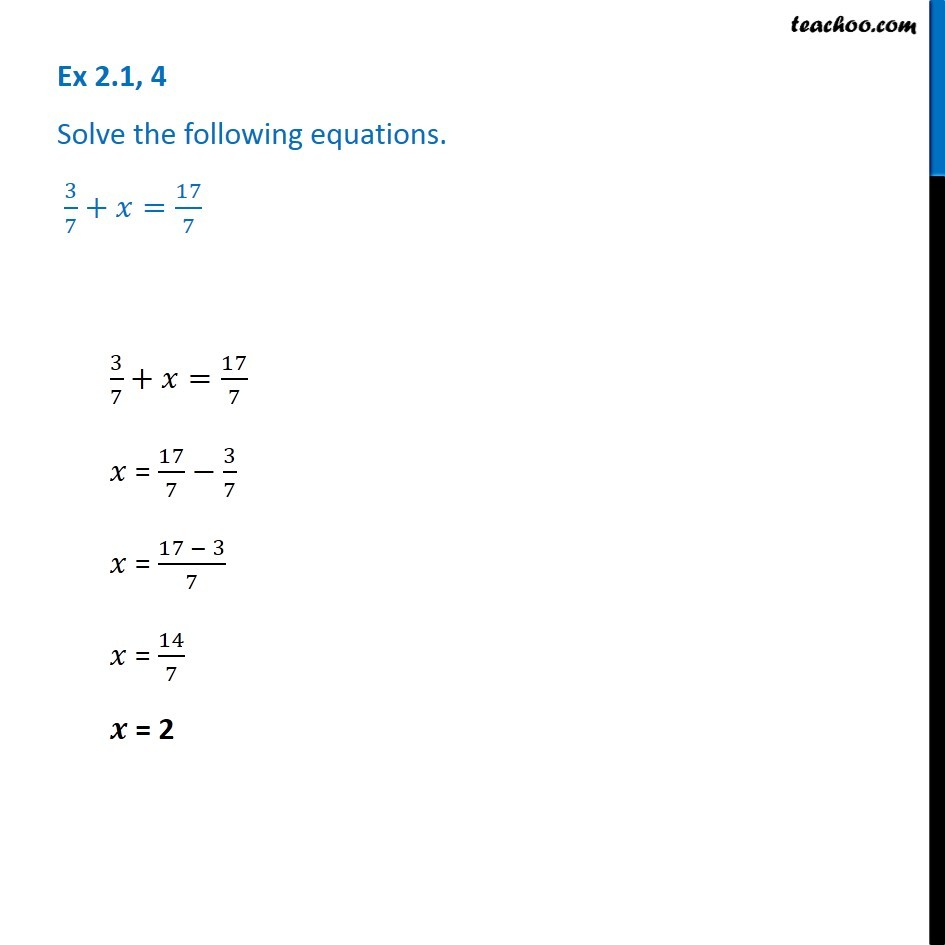Solving easy equations

Chapter 2 Class 8 Linear Equations in One Variable
Serial order wiseLearn in your speed, with individual attention - Teachoo Maths 1-on-1 Class

### Transcript

Question 4 - Chapter 2 Class 8 NCERT - Linear Equations in one variable Solve the following equations. 3/7+ x = 17/7 3/7+ x = 17/7 x = 17/7 - 3/7 x = (17 - 3)/7 x = 14/7 x = 2